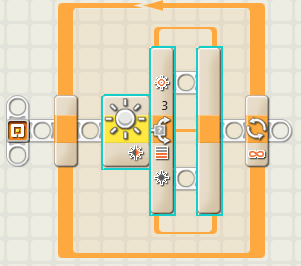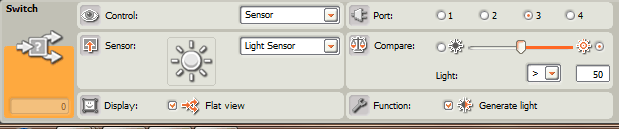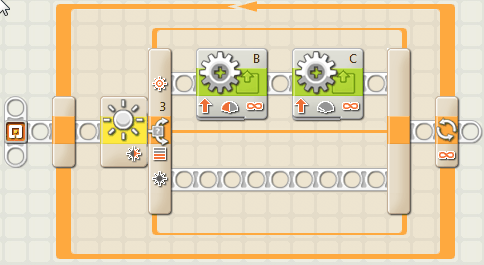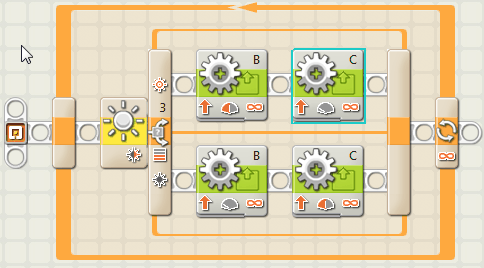LEGO Mindstorms Class:  Lesson 5

Line Following with the Light Sensor:

In the last class we used the Light Sensor to detect when our robot encountered a dark line.  In this class, we're going to use the Light Sensor to help our robots follow along a dark line.  Specifically, we will follow the edge of the line.

Let's say we want our robot to follow a dark line on white paper.  Remember, if we calibrated it, the light sensor will output a value from 0 to 100 depending on how much of the sensor is over the dark line.  0 means the sensor is totally over the line.  100 means the sensor is only over the white paper.  Imagine what the sensor would see if it moved from left to right over a dark line (see picture below):Before we work with the line sensor, we'll need to calibrate it for the lighting conditions in the class today.  Last week you loaded a program onto your robot called "Calibrate.rbt".  Run it by holding the light sensor completely over the black line, and pressing the orange button on the brick, then holding the robot over the white paper and pressing the orange button again.  You can test the calibration by viewing the output of the light sensor in a Light Sensor Block in the NXT-G window.  Ask your instructors for help if you don't remember how to do this.

Exercise 1:  Write a line following program

We're going to tell our robot to follow the left edge of the line.  In the picture above, we see that the sensor reading is high (> 50) if the sensor is mostly over the paper, and the sensor reading is low (< 50) if the sensor is mostly over the line.  We can use this fact to keep the sensor lined up with the left edge of the line while the robot moves forward.  Let's write some pseudocode that does this:

• Get output from the sensor
• If the light sensor output is greater than ( > ) 50, we must be left of the line's edge
• Move forward and turn slightly to the right
• If the output is less than ( < )  50, we must be right of the line's edge
• Move forward and turn slightly to the left
• Repeat from the beginning

Now let's turn this pseudocode into an actual NXT-G program.  Create a program called linefollow.rbt, and save it to your folder.

1. Since the program repeats, we'll need a loop1. Now notice we have an "If" statement in our pseudocode.  This means we have to make a decision based on the output of the light sensor.  We'll use the "Switch" block (from the Common menu) for this.  Select the settings on the switch block to use the light sensor output, and make a decision based on whether it is greater than or less than 50.1. First fill in the top part of the switch statement.  This is what will happen if the light sensor value is greater than 50 (see that the "light symbol" looks bright).  Remember that a value greater than 50 means that the robot is positioned left of the line.  We want to make the robot move forward, and slightly to the right to get closer to the line.  Instead of using a Move Block, we are going to use two Motor Blocks to do this.  The motor blocks give the robot a little more control over the turn, and make the movement smoother.  Notice we have one motor block for motor B, and one for Motor C.  Since Motor B is on the left and Motor C is on the right,  if motor B spins faster (has more power) than Motor C, the robot will move to the right.  Set the power for Motor B to 50 and the power for Motor C to 10.1. Now let's fill in the bottom part of the switch statement.  This is what we do if the light sensor returns a value less than 50.  When the value is less than 50, the light sensor is mostly over the line.  Since we want the robot to follow the left edge of the line, we'll want it to move forward, and slightly to the left.  In this case, we'll want Motor C to have more power than Motor B.  Add two motor blocks like we did in step 3, but set the power for Motor B to 10 and the power for Motor C to 50.That's the whole program!  Your instructor has made a course out of paper and electrical tape.  Try placing the robot near the left edge of the line and seeing if it can follow the course.

Challenges:

Challenge 1:  The need for speed!

Your instructors have set up a course mapped out in electrical tape.  Time how long it takes your robot to complete the course.  Can you think of ways to make it go faster without losing its way?   Hint:  Try completing Challenge 2 below for one method to make your robot a speedier line follower.

Challenge 2: Write a 3-state line following program

The line following program we just wrote works best following a line where the curves aren't too sharp.  If you try to get the robot to follow a sharply angled turn, it may lose the line entirely.  The robot also moves pretty slowly, since it has to spend so much time turning left and right to find the edge of the line.  Let's try a method to make the robot move faster and more accurately.

Instead of telling the robot what to do based on whether it is left or right of the line (two different states), let's make it more sensitive to its position.  We will let the robot travel straight if it is close to the edge of the line, and only turn left or right when it moves away from the edge it is trying to follow.  Since it reacts differently to three different conditions (left of edge, right of edge, close to edge) this is called a 3-State Program.  Here is the pseudocode we want to follow:

• Get output from the sensor
• If the light sensor output is greater than ( > ) 60, we must be left of the line's edge
• Move forward and turn slightly to the right
• Otherwise the light sensor output must be less than (<) 60
• If the light sensor is less than 60 but greater than 40, we must be close to the edge
• Move straight forward
• Otherwise the light sensor is less than 40 and we are right of the line's edge
• Move  forward and turn slightly to the left
• Repeat from the beginning

The code may look tricky because it has an "if" statement inside another "if" statement, but if you think carefully, you will see how to write the program.   You will need to use more than one switch statement to get it to work.  Ask your instructors if you need help.An example of a 3-state line following program.